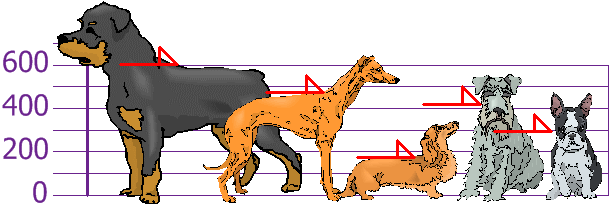# 平均差

## 怎样计算

• 一、求所有数据值的平均值
• 二、求每个数据与平均值的距离（用数据值减去平均值，不理正负号）
• 三、求这些距离的平均

### 例子：3、6、6、7、8、11、15、16 的 平均差

 平均 = 3 + 6 + 6 + 7 + 8 + 11 + 15 + 16 = 72 = 9 8 8

3 6
6 3
6 3
7 2
8 1
11 2
15 6
16 7（没有正负号！）

 平均差 = 6 + 3 + 3 + 2 + 1 + 2 + 6 + 7 = 30 = 3.75 8 8

## 公式

• Σ总和符号，意思是加起来
• || （垂直线）的意思是 绝对值，就是不要正负号
• x 是每个数据值（例如 3 或 16）
• μ 是平均（在这例子里，μ = 9
• N 是数据点的个数（在这例子里，N = 8

## 绝对偏差我们把 "|" 放在项的两变来代表 "绝对值"： |-3| = 3 为每一个 x： 绝对偏差 = |x - μ|

## 总和符号

"加起来" 的符号是 Σ（叫总和符号)，所以：

### 例子： 3、6、6、7、8、11、15、16 的 平均差

 μ = 3 + 6 + 6 + 7 + 8 + 11 + 15 + 16 = 72 = 9 8 8

x |x - μ|
3 6
6 3
6 3
7 2
8 1
11 2
15 6
16 7
Σ|x - μ| = 30

 平均差 = Σ|x - μ| = 30 = 3.75 N 8

## 平均差的意思是什么？

### 例子：你和你的朋友测量了你们的狗的身高（毫米）：μ = 600 + 470 + 170 + 430 + 300 = 1970 = 394 5 5

x |x - μ|
600 206
470 76
170 224
430 36
300 94
Σ|x - μ| = 636

 平均差 = Σ|x - μ| = 636 = 127.2 N 5

（可以和 147 mm标准差比较一下）

## 检测

### 例子：3、6、6、7、8、11、15、166 + 3 + 3 + 2 + 1 = 2 + 6 + 7 15 = 15